Question

# Use the References to access important values if needed for this question moles 1. How many...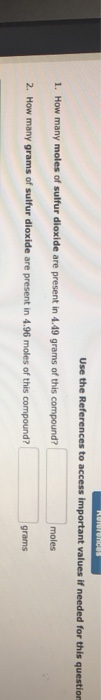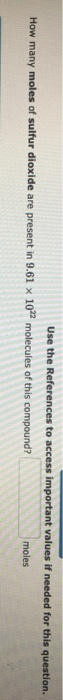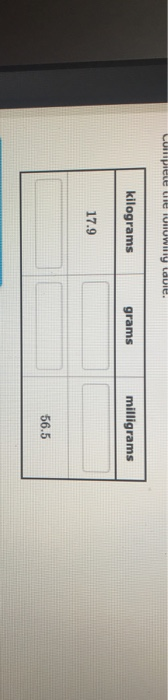Use the References to access important values if needed for this question moles 1. How many moles of sulfur dioxide are present in 4.49 grams of this compound? 2. How many grams of sulfur dioxide are present in 4.96 moles of this compound grams
Use the References to access important values if needed for this question How many moles of sulfur dioxide are present in 9.61 × 1022 molecules of this compound? moles
kilograms grams milligrams 17.9 56.5

We need at least 10 more requests to produce the answer.

0 / 10 have requested this problem solution

The more requests, the faster the answer.

All students who have requested the answer will be notified once they are available.

#### Earn Coins

Coins can be redeemed for fabulous gifts.

Similar Homework Help Questions
• ### Use the References to access important values if needed for this question. How many ATOMS of...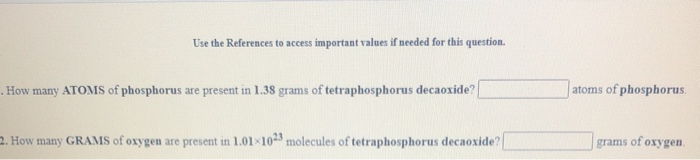Use the References to access important values if needed for this question. How many ATOMS of phosphorus are present in 1.38 grams of tetraphosphorus decaoxide? atoms of phosphorus. 2. How many GRAMS of oxygen are present in 1.01 1023 molecules of tetraphosphorus decaoxide? grams of oxygen days grams 1. How many GRAMS of potassium sulfate are present in 4.96 moles of this compound? moles 2. How many MOLES of potassium sulfate are present in 1.67 grams of this compound?

• ### Use the References to access important values if needed for this question. 1. How many MOLECULES...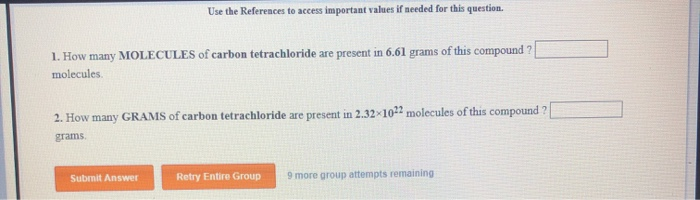Use the References to access important values if needed for this question. 1. How many MOLECULES of carbon tetrachloride are present in 6.61 grams of this compound? molecules 2. How many GRAMS of carbon tetrachloride are present in 2.32 1022 molecules of this compound? grams Submit Answer Retry Entire Group 9 more group attempts remaining

• ### Use the References to access important values if needed for this question. 1. How many ATOMS...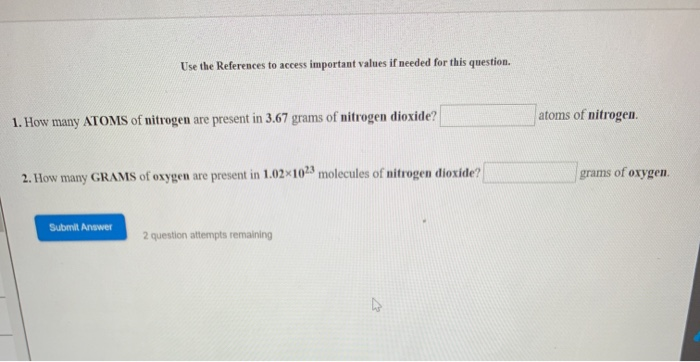Use the References to access important values if needed for this question. 1. How many ATOMS of nitrogen are present in 3.67 grams of nitrogen dioxide? atoms of nitrogen 2. How many GRAMS of oxygen are present in 1.02x102) molecules of nitrogen dioxide? grams of oxygen Submit Answer 2 question attempts remaining

• ### Use the References to access important values if needed for this question. 1. How many GRAMS...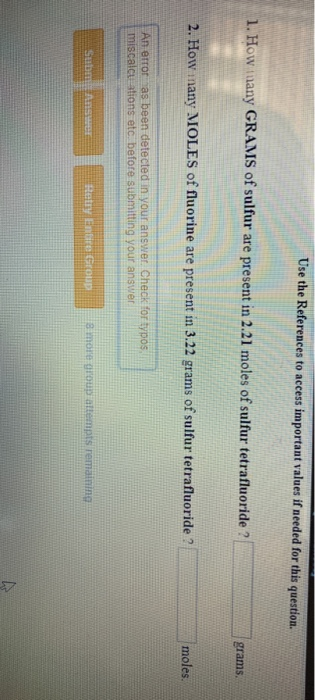Use the References to access important values if needed for this question. 1. How many GRAMS of sulfur are present in 2.21 moles of sulfur tetrafluoride ? grams. 2. How inany MOLES of fluorine are present in 3.22 grams of sulfur tetrafluoride ? moles. An error as been detected in your answer. Check for typos miscalcuations etc, before submitting your answer Hetry Entire Group SEHOLD lemn

• ### Use the References to access important values if needed for this question. a. How many grams...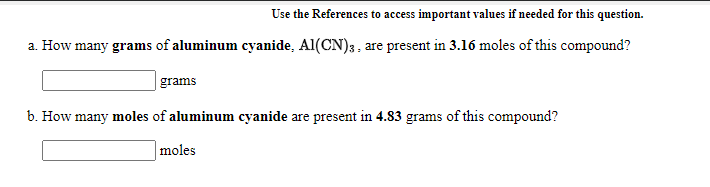Use the References to access important values if needed for this question. a. How many grams of aluminum cyanide. Al(CN)3, are present in 3.16 moles of this compound? grams b. How many moles of aluminum cyanide are present in 4.83 grams of this compound? moles

• ### Use the References to access important values if needed for this question. 1. How many ATOMS...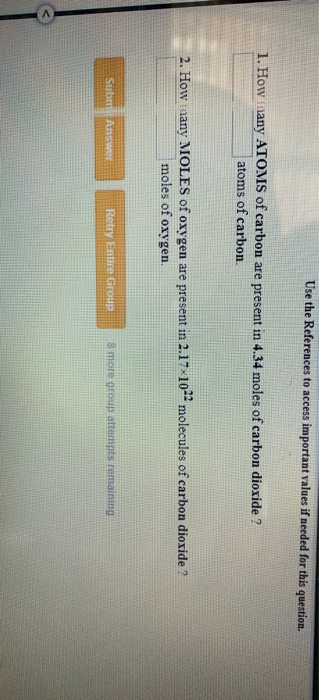Use the References to access important values if needed for this question. 1. How many ATOMS of carbon are present in 4.34 moles of carbon dioxide ? atoms of carbon 2. How many MOLES of oxygen are present in 2.17x10-- molecules of carbon dioxide ? moles of oxygen SUDIT ARS Retry Entre Group group attempts remaining

• ### Use the References to access important values if needed for this question. a. How many grams...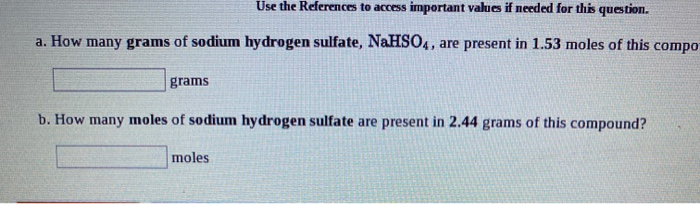Use the References to access important values if needed for this question. a. How many grams of sodium hydrogen sulfate, NaHSO4, are present in 1.53 moles of this compo grams b. How many moles of sodium hydrogen sulfate are present in 2.44 grams of this compound? moles

• ### Use the References to access important values if meeded for this question molecules 1. How many...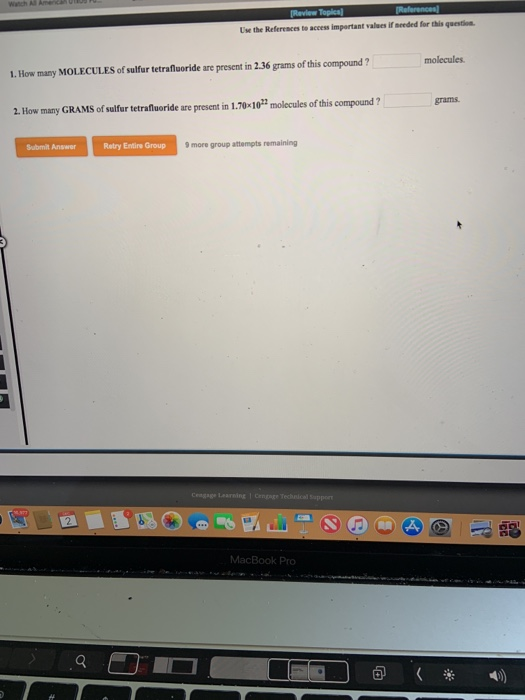Use the References to access important values if meeded for this question molecules 1. How many MOLECULES of sulfur tetrafluoride are present in 2.36 grams of this compound? grams 2. How many GRAMS of sulfur tetrafiuoride are present in 1.70-10t molecules of this compound? Submit Answer Retry Entire Group 9 more group attempts remaining Learning 諨

• ### Use the References to access important values if needed for this question a. How many grams...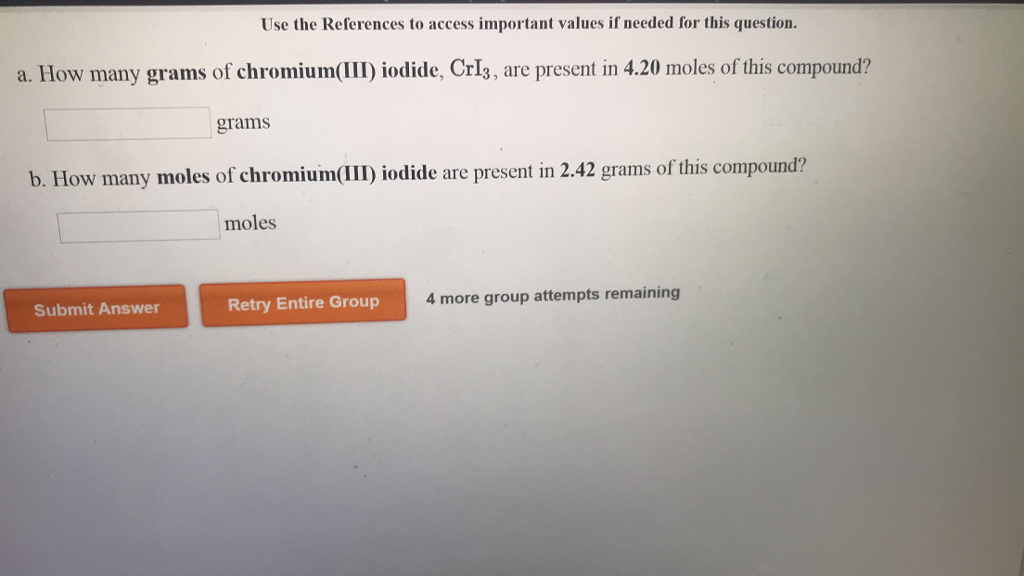Use the References to access important values if needed for this question a. How many grams of chromium(I) iodide, CrI3, are present in 4.20 moles of this compound? grams b. How many moles of chromium(III iodide are present in 2.42 grams of t moles Submit Answer Retry Entire Group 4 more group attempts remaining

• ### References Use the References to access important values if needed for this question. According to the...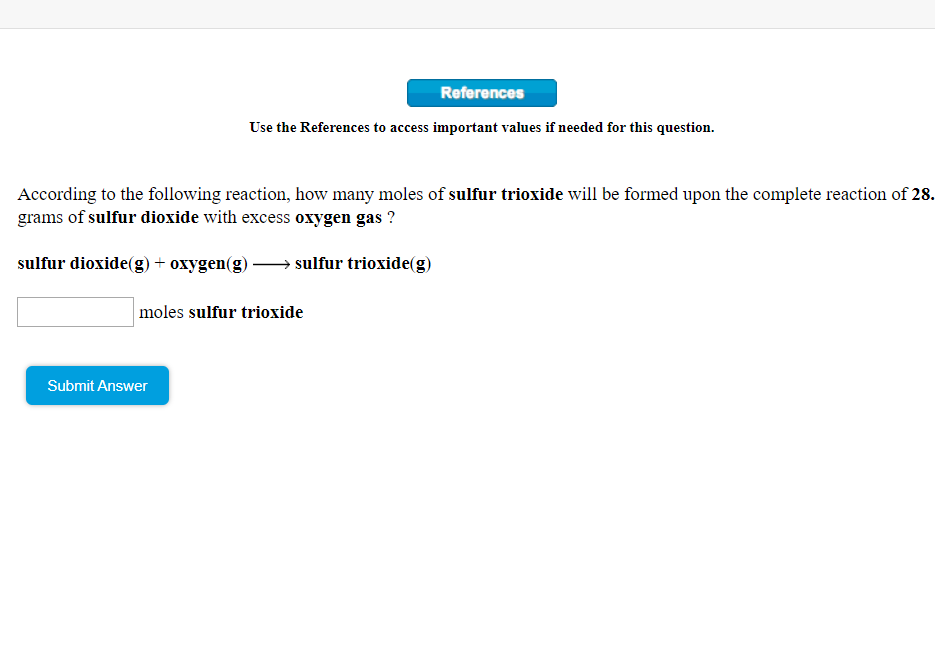References Use the References to access important values if needed for this question. According to the following reaction, how many moles of sulfur trioxide will be formed upon the complete reaction of 28. grams of sulfur dioxide with excess oxygen gas ? sulfur dioxide(g) + oxygen(g) sulfur trioxide(g) moles sulfur trioxide Submit Answer Q Searc References Use the References to access important values if needed for this question. According to the following reaction, how many moles of sulfur trioxide will...